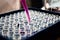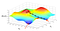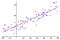# Introduction

We will be discussing about Naive Bayes Classifier in this post as a part of Classification Series. First, we will look at what Naive Bayes Classifier is, little bit of math behind it, which applications are Naive Bayes Classifier typically used for, and finally an example of SMS Spam Filter using Naive Bayes Classifier.

## What is Naive Bayes Classifier ?

where A and B are events and P ( B ) ≠ 0.

• P ( A ∣ B )…

# Introduction

In this post we will be exploring and understanding one of the basic Classification Techniques in Machine Learning — Logistic Regression.

Originally published at https://machinelearningmind.com on November 25, 2019.

• Binary logistic regression: It has only two possible outcomes. Example- yes or no
• Multinomial logistic regression: It has three or more nominal categories. Example- cat, dog, elephant.
• Ordinal logistic regression- It has three or more ordinal categories, ordinal meaning that the categories will be in a order. Example- user ratings(1–5).

For now, lets focus on Binary Logistic Regression.

# Introduction

I will assume that you have a fair understanding of Linear Regression. If not, I have written a simple and easy to understand post with example in python here. Read it before continuing further.

Linear Regression makes certain assumptions about the data and provides predictions based on that. Naturally, if we don’t take care of those assumptions Linear Regression will penalise us with a bad model (You can’t really blame it!)
We will take a dataset and try to fit all the assumptions and check the metrics and compare it with the metrics in the case that we hadn’t worked on…

# Multicollinearity — How to fix it?

This post will answer questions like What is multicollinearity? What are the problems that arise out of Multicollinearity? When do I have to fix Multicollinearity? and How to fix Multicollinearity?

One of the important aspect that we have to take care of while regression is Multicollinearity. Check this post to find an explanation of Multiple Linear Regression and dependent/independent variables.

Just a refresher,

• Dependent variable is the one that we want to predict.
• Independent variable is the one that is used to predict the dependent variable.
• Our goal in regression is to find out which of the independent variables can…

# Feature Elimination Using p-valuesPhoto by Louis Reed on Unsplash

# Introduction

In this post we will be talking about Hypothesis Testing, How p-value is calculated and how it helps us in Hypothesis Testing with a simple example of Students’ scores . Then we will move on to how it helps us in eliminating Features in a Medical Expenses Dataset, and then fit a model to it.

# P-values

In statistical hypothesis testing, the p- value or probability value is the probability of obtaining test results at least as extreme as the results actually observed during the test, assuming that the null hypothesis is correct.

Wikipedia

Ok! let’s break that down. Now some of…

# Gradient Descent — Intro and Implementation in pythonGradient Descent in action

# Introduction

Gradient Descent is an optimization algorithm in machine learning used to minimize a function by iteratively moving towards the minimum value of the function.

We basically use this algorithm when we have to find the least possible values that can satisfy a given cost function. In machine learning, more often that not we try to minimize loss functions (like Mean Squared Error). By minimizing the loss function , we can improve our model, and Gradient Descent is one of the most popular algorithms used for this purpose.

# Introduction To Linear Regression — E-commerce DatasetLinear Regression Model

In this post , we will be understanding what Linear Regression is, A little bit of the math behind it and try to fit a Linear Regression model on an E-commerce Dataset.

# Linear Regression

Wikipedia says ‘..linear regression is a linear approach to modeling the relationship between a scalar response (or dependent variable) and one or more explanatory variables (or independent variables). The case of one explanatory variable is called simple linear regression. For more than one explanatory variable, the process is called multiple linear regression.

In more layman terms, Linear regression model is used to predict the relationship between variables or…## FAHAD ANWAR

Data Science | AI | Big Data | Machine Learning | Python | www.linkedin.com/in/fahad-anwar10 | www.machinelearningmind.com